## FORM THREE MATHEMATICS STUDY NOTES TOPIC 2: FUNCTIONS

### FORM THREE MATHEMATICS STUDY NOTES TOPIC 2: FUNCTIONSMonday, April 6, 2020

FORM THREE MATHEMATICS STUDY NOTES TOPIC 2: FUNCTIONS

UNAWEZA JIPATIA NOTES ZETU KWA KUCHANGIA KIASI KIDOGO KABISA:PIGA SIMU:0787237719

## FORM THREE MATHEMATICS STUDY NOTES TOPIC 2: FUNCTIONS

FUNCTIONS

Normally relation deals with matching of elements from the first set called DOMAIN with the element of the second set called RANGE.
Definitions:
A function is a relation with a property that for each element in the domain there is only one corresponding element in the range or co- domain
Therefore functions are relations but not all relations are functions
The Concept of a Functions Pictorially
Explain the concept of a functions pictorially
Example 1
Which of the following relation are functions?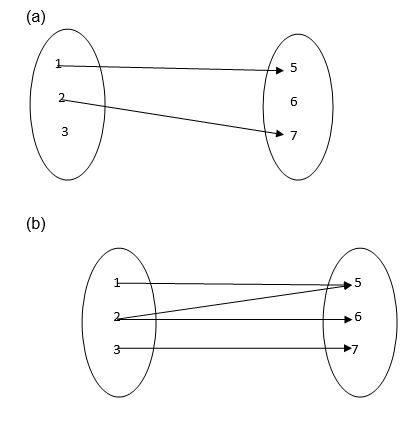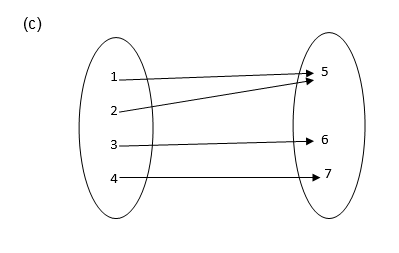Solution
1. It is not a function since 3 and 6 remain unmapped.
2. It is not a function because 2 has two images ( 5 and 6)
3. It is a function because each of 1, 2, 3 and 4 is connected to exactly one of 5, 6 or 7.

Functions
Identify functions
TESTING FOR FUNCTIONS;
If a line parallel to the y-axis is drawn and it passes through two or more points on the graph of the relation then the relation is not a function.
If it passes through only one point then the relation is a function
Example 2
Identify each of the following graphs as functions or not.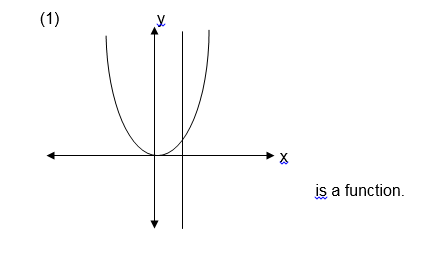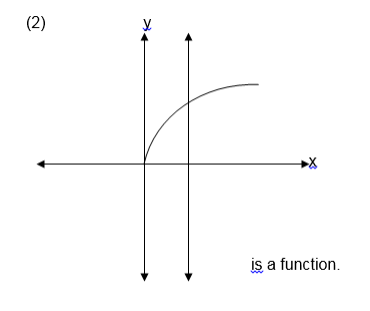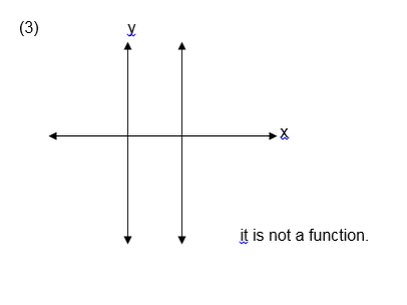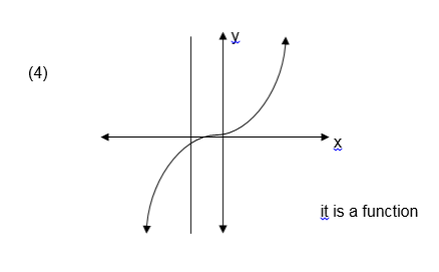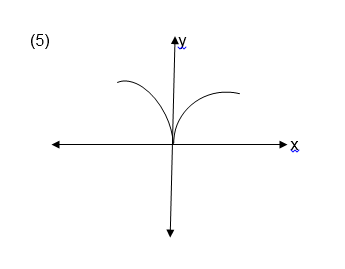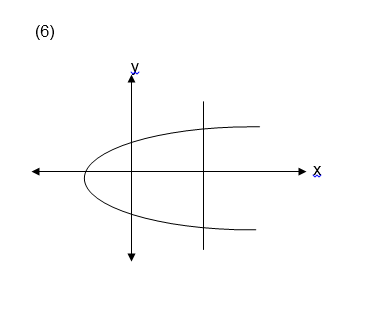Exercise 1
1. Which of the following relations are functions?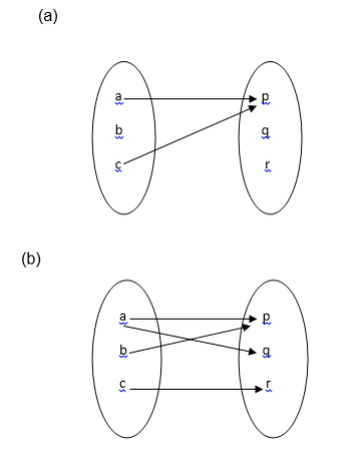2. Let A = {1, 2, 3, 4, 5, 6, 7, 8, 9, 10}
and B ={ 2, 3, 5, 7 }
Draw an arrow diagram to illustrate the relation “ is a multiple of ‘ is it a function ? why?
3. let A = {1,-1 ,2,-2} and
B = {1, 2, 3, 4 } which of the following relations are functions ?
1. { ( x , y ) : x < y }
2. { ( x , y ) : x > y}
3. { ( x , y ) : y = x2}
FORM THREE MATHEMATICS STUDY NOTES TOPIC 2: FUNCTIONS
4/ 5
Oleh